# Moneys in triple ratio

Milan, John and Lili have a total 344 euros. Their amounts are in the ratio 1:2:5. Determine how much each of them has?

m =  43 Eur
j =  86 Eur
l =  215 Eur

### Step-by-step explanation: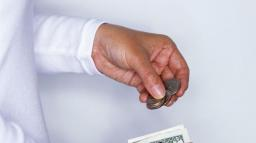Did you find an error or inaccuracy? Feel free to write us. Thank you!Tips to related online calculators
Check out our ratio calculator.

## Related math problems and questions:

• AgesJohn, Teresa, Daniel and Paul have summary 56 years. Their ages are in a ratio of 1:2:5:6. Determine how many years have each of them.
• Ratio6 numbers are in the ratio 1:5:1:5:5:5. Their sum is 242. What are the numbers?
• Division of moneyCalculate how many euros have Matthew, Miriam, Lucy, Michael, Janka when together have 2,700 euros and the amounts are at a ratio of 1:5:6:7:8.
• Dividing moneyMilan divided 360 euros in the ratio 4: 5, Erik in the ratio 500: 625. How many euros did the individual parts of Milan and how many Erik?
• Money split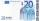Anton and Ferko got 2,500 euros together. The amount was split in a 1:4 ratio. How much did each get?
• Divide moneyDivide 1200 USD at a ratio of 1:2:3:4:5:6:9:10
• Ratio three numbers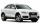Three numbers SUV are in the ratio 1:2:3. Their sum is 24. Find this numbers and write their add and sum.
• Honza + Alice + Tonda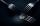Honza + Alice + Tonda have a total of 111 USD. The ratio between Honza and Alena is 5:6 and the ratio between Alice and Tonda is 4:5 How many money have each of them?
• Euros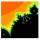Michal, Peter, John and Lenka got together 2,400 euros. They share an amount in ratio 2:6:4:3. How many got each of them?
• ErikThree friends Peter, Erik and Milan shared 420 euros in a ratio of 1: 2: 3. How many euros did Erik get?
• RatiosReduce the numbers: 50 in a 1:2 ratio 111 at a ratio of 2:3 70 at 10:50 560 at a ratio of 3:8
• BuildingAt the building, we divided 240 boards into two piles in a 5: 3 ratio. How many were fewer boards in the lower pile?
• Land cost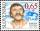The land is worth 33295 CZK. 1st owner has 5/15, 2nd owner has 4/15, 3rd owner has 1/5 4th owner has 1/5. How much CZK each of them will receive?
• Report 2A School reports students to teacher ratio of 6:1. If there are 45 teachers in the School, how many students are there?
• PeppersIn the box are yellow (a), green (b), and red (c) peppers. Their amount is in a ratio of 2:4:1. Most are yellow peppers and green the least. Calculate the number of peppers each type if the total number of peppers is 70.
• Fractions and mixed numerals(a) Convert the following mixed numbers to improper fractions. i. 3 5/8 ii. 7 7/6 (b) Convert the following improper fraction to a mixed number. i. 13/4 ii. 78/5 (c) Simplify these fractions to their lowest terms. i. 36/42 ii. 27/45 2. evaluate the follow
• RemaindersIt is given a set of numbers { 170; 244; 299; 333; 351; 391; 423; 644 }. Divide this numbers by number 66 and determine set of remainders. As result write sum of this remainders.